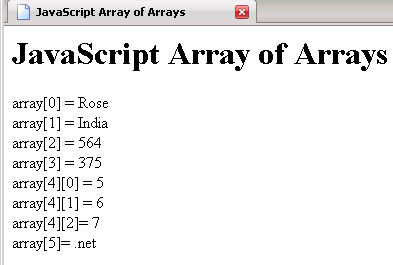Tutorials

# JavaScript array of arrays

In this Tutorial we want to describe you a code that help you in understanding JavaScript array of arrays.

In this Tutorial we want to describe you a code that help you in understanding JavaScript array of arrays.

# JavaScript array of arrays

In this Tutorial we want to describe you a code that help you in understanding JavaScript array of arrays. For this we are using JavaScript as Script language. We instantiate an array object by passing argument and object of array to it and allocate them to the memory. The element in the array can be accessed by its numerical index. Finally the document. write print the list of  element in an array.

javascriptArrayofArray.html

 ``` JavaScript Array of Arrays

JavaScript Array of Arrays

var array = new Array("Rose","India",564,375,new Array(5,6,7),".net") document.write("array = "+array+"
") document.write("array = "+array+"
") document.write("array = "+array+"
") document.write("array = "+array+"
") document.write("array = "+array+"
") document.write("array = "+array+"
") document.write("array= "+array+"
") document.write("array= "+array+"
") ```

Output of the program